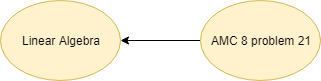Categories

# Linear Equation AMC 8 2010 problem 21

Try this beautiful problem from AMC 8. It involves the concept that when a number is taken as a fraction. And also based upon the basic Linear Equation calculation.

# What are we learning ?

Competency in Focus: Linear Equation This problem from American Mathematics contest (AMC 8, 2010 problem 21) is based on Linear Equation

# First look at the knowledge graph.# Next understand the problem

Hui is an avid reader. She bought a copy of the best seller Math is Beautiful. On the first day, Hui read $\frac{1}{5}$ of the pages plus 12 more, and on the second day she read 1/4 of the remaining pages plus 15 pages. On the third day she read 1/3 of the remaining pages plus 18 pages. She then realized that there were only 62 pages left to read, which she read the next day. How many pages are in this book? (A) 120  (B) 180  (C) 240  (D) 300  (E) 240
##### Source of the problem
American Mathematical Contest 2010, AMC 8 Problem 21
##### Key Competency
This number theory problem is based on the concept of linear equation
5/10
##### Suggested Book
Challenges and Thrills in Pre College Mathematics Excursion Of Mathematics

Do you really need a hint? Try it first!
You can assume total number of pages to be a variable say x.
Every next day she is reaing a fraction of the REMAINING pages, not the total pages.
she also reads some more pages on each day, so we need to subtract this also to get the actual remaining page
At the en of third day total remining page in term of x must be 62 as state in the question.

# Connected Program at Cheenta

#### Amc 8 Master class

Cheenta AMC Training Camp consists of live group and one on one classes, 24/7 doubt clearing and continuous problem solving streams.

# Similar Problems

## One reply on “Linear Equation AMC 8 2010 problem 21”

first day he reads x pages;
second day he reads y pages;
third day he reads z pages;
there are 62 pages are remaining;
so,Total no of pages= (x+y+z+62) pages;
According to question, x= (x+y+z+62)/5+12;
y=(y+z+62)/4+15;
z= (z+62)/3+18;
solving them,we get x=58;y=60;z=60;
So,Total no of pages in the Book=(x+y+z+62) pages= (58+60+60+62) pages=240 pages OPTION (C)

This site uses Akismet to reduce spam. Learn how your comment data is processed.